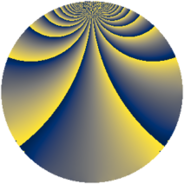# Properties

 Label 1386.2.bqLevel $1386$ Weight $2$ Character orbit 1386.bq Rep. character $\chi_{1386}(125,\cdot)$ Character field $\Q(\zeta_{10})$ Dimension $128$ Sturm bound $576$

# Related objects

## Defining parameters

 Level: $$N$$ $$=$$ $$1386 = 2 \cdot 3^{2} \cdot 7 \cdot 11$$ Weight: $$k$$ $$=$$ $$2$$ Character orbit: $$[\chi]$$ $$=$$ 1386.bq (of order $$10$$ and degree $$4$$) Character conductor: $$\operatorname{cond}(\chi)$$ $$=$$ $$231$$ Character field: $$\Q(\zeta_{10})$$ Sturm bound: $$576$$

## Dimensions

The following table gives the dimensions of various subspaces of $$M_{2}(1386, [\chi])$$.

Total New Old
Modular forms 1216 128 1088
Cusp forms 1088 128 960
Eisenstein series 128 0 128

## Trace form

 $$128q + 32q^{4} + 8q^{7} + O(q^{10})$$ $$128q + 32q^{4} + 8q^{7} - 32q^{16} - 24q^{25} + 12q^{28} + 32q^{37} + 32q^{43} + 16q^{46} + 8q^{49} - 40q^{58} + 32q^{64} + 128q^{67} + 4q^{70} - 80q^{79} + 8q^{85} + 32q^{91} + O(q^{100})$$

## Decomposition of $$S_{2}^{\mathrm{new}}(1386, [\chi])$$ into newform subspaces

The newforms in this space have not yet been added to the LMFDB.

## Decomposition of $$S_{2}^{\mathrm{old}}(1386, [\chi])$$ into lower level spaces

$$S_{2}^{\mathrm{old}}(1386, [\chi]) \cong$$ $$S_{2}^{\mathrm{new}}(231, [\chi])$$$$^{\oplus 4}$$$$\oplus$$$$S_{2}^{\mathrm{new}}(462, [\chi])$$$$^{\oplus 2}$$$$\oplus$$$$S_{2}^{\mathrm{new}}(693, [\chi])$$$$^{\oplus 2}$$